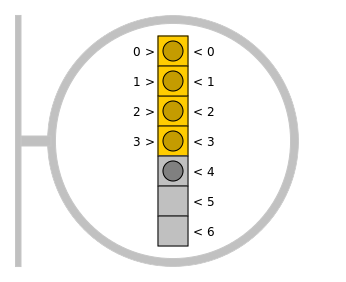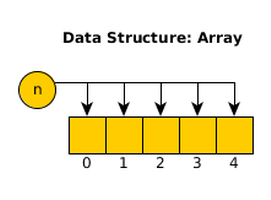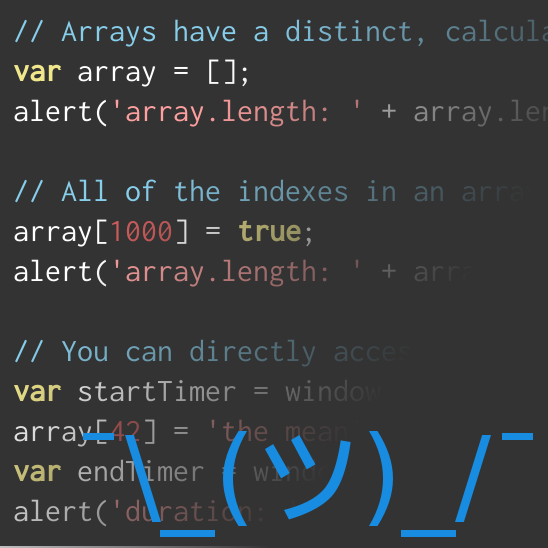## Array Lists: A Less Awful Way to Handle ArraysDifferent languages use different approaches when implementing the array data structure, with some wide variation between them. In JavaScript, for example, arrays are exceptionally simple to work with; while the C++ implementation can be a comparative nightmare. You're given a limited number of slots to work with, the only way to reindex is to manually copy elements between those slots, and there's no convenient and consistent way to extract an array's length from the structure. It's a lot of work to keep all of this information straight, but sometimes you just really need to be able to access the 1-billionth element in an array and a $\Theta(1)$ runtime is far more attractive than $\Theta(n)$.

This is where the Array List data structure stands out. An Array List is a derivative of the List pattern that can be used as a drop-in replacement for any other List structure, but which uses an array as the data repository. This integration of an array in the data structure gives it a very different performance profile than the Linked List; and giving it a very different optimal use-case.

### Storing Data

It may seem obvious, but the first thing our data structure must be able to do is to exist with the capability of storing data. The challenge we are facing is in how we are going to map the expectations of the external code to the array in such a way that functions could be written to receive either an ArrayList or a LinkedList object without being opinionated about how either should be used. We already know that we need to store content in an array. We also know how external code must be able to interact with our list.

template<typename T>
class ArrayList : ArrayListInterface<T>
{
public:
ArrayList()
{
this->array = new T;
}

~ArrayList()
{
// Destruct
}
protected:
T* array;
};

These constraints allow us to obscure the array so that it cannot be directly accessed. This will help protect our data structure from meddling or clumsy edits.

Since our array uses explicit indexes to track data, we have some additional work to do before we can start building the public interface. First, we need to determine how we are going to differentiate between the outward-facing "logical length" of the data structure, and the internal "physical length" of the data structure. Second, we need to decide how we are going to handle the inevitable case of when the logical length extends beyond the physical length of the array. Finally, we need to determine how we are going to reindex elements during an insertion or deletion.

#### Logical vs. Physical Length

We can solve the first problem by creating two internal variables. The first will track the physical length of the array, and the second will track the logical length of the array:

public:
ArrayList() : logicalLength(0), physicalLength(16)
{
this->array = new T[this->physicalLength];
}
// ...
protected:
unsigned int logicalLength;
unsigned int physicalLength;

Our "Logical Array" will be the array we are pretending to have in memory, while our "Physical Array" will be the array we actually have in memory. By differentiating between the two arrays, we can write a more efficient array structure that follows the List schema without incurring the performance costs of constantly extending or contracting an array.

#### Extending the Array

Tracking both the physical and logical lengths of the array gives us a clear method for determining when the logical array is outgrowing the physical array. We can take advantage of this to create a function that extends the physical array for us.

One problem: copying an array gets more expensive the larger the array gets. This grows at a rate of $\Theta(n)$, and so we'll need an offset to keep that from slowing our data structure down. I've decided to offset this by doubling the size of the array each time we need to extend it. This prevents us from having to use an absurdly large array from the very start, and it ensures that the frequency of extending an array comes in at $O(log_2(n))$. This isn't a perfect solution, but it should serve our purposes well enough, as this function will be called fewer times as the impact of the execution grows.

ArrayList<T>& extendArray()
{
// double the size of the array
this->physicalLength <<= 1;

T* array = new T[this->physicalLength];
for (unsigned int i = 0; i < this->logicalLength; ++i) {
array[i] = this->array[i];
}
this->array = array;

return *this;
}

#### Reindexing the Array

The final challenge we need to resolve before our list is complete is in handling reindexes. In an array of $n$ elements, inserting or removing an index $i$ to/from the array requires every index from $i$ through $n$ to be updated; an operation that incurs an $O(n)$ cost.

For this implementation, I've chosen to take the simple route. If we remove an element, then we will shift array contents to the left to fill the void. Likewise, if we insert an element, then we will shift array contents to the right to create a void.

### Filling in the Structure

Now that we've figured out the esoterica of an Array List versus the generic List implementation, the next step is to figure out how to implement the individual insert, remove, get, set, and count functions.

#### Inserting Values

Our Array List needs to be consistent with the List interface. To do this, we need a way to insert elements at specific indexes, or else at the end of the array. When inserting an element into the data structure, we will need to update the logical length of the array, ensure that we have enough space in the array, and reindex any shifted elements. Luckily, we've already decided how to handle all of this.

However, we also need to maintain some degree of consistency with the Linked List class. Although we are technically allowed to insert elements into the indexes between the logical length of the array and the physical length of the array, we should not allow this behavior. To prevent it, we will throw an error whenever anything is explicitly requested beyond the logical length of the array.

ArrayList<T>& insert(T value)
{
return this->insert(value, this->logicalLength);
}

ArrayList<T>& insert(T value, unsigned int index)
{
// make sure we're in-bounds
if (index > this->logicalLength) {
throw new std::out_of_range("Expected index to be within length of list");
}

// extend the array if necessary
if (1 + this->logicalLength >= this->physicalLength) {
this->extendArray();
}

// create a void
for (unsigned int i = this->logicalLength + 1; i > index; --i) {
this->array[i] = this->array[i - 1];
}

// insert our value
this->array[index] = value;

// increment the logical length
++this->logicalLength;

return *this;
}

In order to read from the data structure, we need to pass it an index to access. Although we could technically access any element inside of our physical array, we also need to limit access here to only the values inside of the logical array. Any index that is out-of-bounds should throw an error, while any other value should return the value at that index in the array:

T get(unsigned int index)
{
if (index >= this->logicalLength) {
throw new std::out_of_range("Expected index to be within length of list");
}

return this->array[index];
}

This function takes full advantage of the array's ability to randomly access any element in the array. The check incurs a minor overhead, but the overall performance of the function rates at $\Theta(1)$. Compare this to the $\Theta(n)$ of the Singly Linked List and you will see why this is a benefit.

#### Updating Values

In order to update an element, we must provide an index to edit and a value to insert. As we established earlier, we can only access elements that occupy indexes within the logical array. If we try to access an element outside of this range, then we'll throw an error.

ArrayList<T>& set(unsigned int index, T value)
{
if (index >= this->logicalLength) {
throw new std::out_of_range("Expected index to be within length of list");
}

this->array[index] = value;

return *this;
}

Like the get function, set takes advantage of the speed of an array, providing a performance of $\Theta(1)$. This is, again, in contrast to the $\Theta(n)$ of the Linked List.

#### Deleting Values

Deleting a value from the array requires a reindexing of the array once the change is made. This process incurs a $\Theta(n)$ cost, compared to the $\Theta(1)$ cost incurred in a Linked List. To complete this task, we need to know which indexes to remove from the array, and shift elements over to obscure those values. Since we can't reliably remove values from an array without the threat of prematurely deleting them from memory, we will let the logical array obscure them from access and then overwrite them as necessary.

We also need to bring the external behavior of the function into compliance with the expectations set by the linked list. If we try to delete elements from the array that exist outside of the array, then we should throw an error to avoid a potential segmentation fault.

ArrayList<T>& remove(unsigned int index, unsigned int count = 1)
{
if (index + count > this->logicalLength) {
throw new std::out_of_range("Expected segment to be within length of list");
}

for (unsigned int i = index; i < this->logicalLength; ++i) {
this->array[i] = this->array[i + count];
}

this->logicalLength -= count;
return *this;
}

#### Counting Values

We only need to create a way to retrieve the logical length of the array to make it easier to iterate. It makes our jobs a little more complicated to increment and decrement this value manually in the insert and delete functions, but it makes this function very straightforward with a $\Theta(1)$ cost:

unsigned int count()
{
return this->logicalLength;
}

### Put it All Together

We can put all of this code together to run the ArrayList through the same tests as the Linked List. By doing so, we can demonstrate that the Linked List and the Array List are functionally interchangeable, even if they have different strengths and weaknesses.

ArrayList<std::string> list;
list.insert("world").insert("hello", 0);
std::cout << list.get(0) << " " << list.get(1) << "!" << std::endl;
std::cout << list.remove(1).get(0) << "!" << std::endl;
std::cout << "count: " << list.count() << std::endl;
std::cout << list.set(0, "goodbye!").get(0) << "!" << std::endl;

Even though the ArrayList and LinkedLists can be used interchangeably, their respective performance differences mean that you probably shouldn't do it. The Linked List will outperform the Array List when reindexing large data sets because nodes only have logical indexes; but they also incur a massive overhead from accessing nodes that aren't explicitly tracked, since all reads require iteration. By contrast, the Array List has a massive edge on the Linked List in terms of random access, so long as it doesn't need to insert or remove indexes from the middle or start of the list. Keep these differences in mind, and you'll be able to apply each structure in a way that best utilizes their respective strengths and mitigates their respective weaknesses.

### Related Blog PostsData Structure: Array